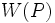# Equivalence of definitions of characteristic p-functor whose normalizer generates whole group with p'-core

This article gives a proof/explanation of the equivalence of multiple definitions for the term characteristic p-functor whose normalizer generates whole group with p'-core
View a complete list of pages giving proofs of equivalence of definitions

## Statement

Suppose$G$ is a group,$p$ is a prime number, and$W$ is a characteristic p-functor. The following are equivalent:

1. For one (and hence every)$p$-Sylow subgroup$P$ of$G$,$O_{p'}(G)N_G(W(P)) = G$
2. For one (and hence every)$p$-Sylow subgroup$P$ of$G$, the image of$W(P)$ in the quotient$G/O_{p'}(G)$ is a normal subgroup of$G/O_{p'}(G)$.
3. For one (and hence every)$p$-Sylow subgroup$Q$ of$K = G/O_{p'}(G)$,$W(Q)$ is a normal subgroup of$K$.
4. For one (and hence every)$p$-Sylow subgroup$Q$ of$K = G/O_{p'}(G)$,$W(Q)$ is a characteristic subgroup of$K$.

## Proof

### Equivalence of (1) and (2)

This is direct from Fact (1). Note that that only uses the conjugacy functor.

### Equivalence of (2) and (3)

This requires the observation that for the quotient map$G \to G/O_{p'}(G)$, Sylow subgroups of$G$ map isomorphically to Sylow subgroups of$G/O_{p'}(G)$, so the quotient map commutes with$W$.

### Equivalence of (3) and (4)

This is direct from Fact (2).